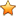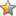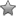# Word Crossy Energy Level 1593 answers!

Welcome! We have all the answers and cheats you need to beat every level of Word Crossy, the addictive game for Android, iPhone, iPod Touch and iPad. This is a game for the true Word Genius! The app game is easy to start and progressively becomes more difficult, making it fun and challenging to play. Connect letters in a level and finish a word. Do it all in the correct way and you will be able to clear the grid. If you don't know the answer use the form below, select your level pack and we will show you all the answers you wanna know. Have fun!
Answers updated: 2018-07-26

The answer to Word Crossy level 1593 is:

D A R K E N

R A N K E D

D R A K E

K N E A D

N A K E D

R A K E D

D A N K

D A R E

D A R K

D A R N

D E A N

D E A R

E A R N

N E A R

N E R D

R A K E

R A N K

R E A D

R E N D

D R A N K

Extra words:

d a n k e r

n a r k e d

k e r n

n a r k

a n d

r a d

r a n

a r e

a r k

e n d

r e d

k e n

k e a

e a r

e r a

d e n(176 votes, average: 2,70 out of 5)Loading...

0.01